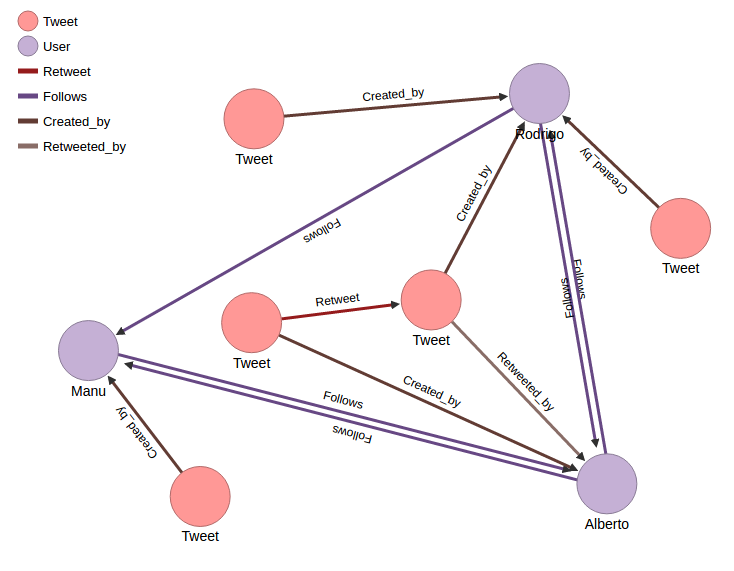# Metrics Usage¶

## Searching for Relevant Tweets and Users¶

Suppose we have 3 twitter users: Manu, Alberto and Rodrigo. This three users are expressing their opinions about a concrete topic in Twitter, i.e about nuclear energy. They have published tweets concerning this topics and they follow each other as it is shown in the following graph.(This data can easily be loaded in Scaner tool using “populate_db.py” script).

## Tweet Ratio¶

To calculate Tweet ratio of each user Scaner takes the lapse of time of the tweets data introduced and relations the number of tweets published in that lapse with the number of tweets related to the topic that are in the Scaner database.

Let’s calculate Rodrigo’s tweet ratio. To do so we choose two intervals of time to measure (t0 y t1). In t0 Rodrigo had 300 tweets in his timeline. In t1 Rodrigo had 333 tweets. Of this 33 new tweets, 3 of them are related to nuclear energy topic and that is the data in Scaner. So the tweet Ratio was:

```import numpy as np
```
```statuses_count_t0 = 300
statuses_count_t1 = 333
tweets_in_scaner = 3

#TWEET RATIO
TR = tweets_in_scaner/(statuses_count_t1-statuses_count_t0)
TR
```
```0.09090909090909091
```

## Influence¶

To find out the influence of users and tweet in this data we need to create three matrix: on one hand with the number of tweets created by the users, on the other, with the retweets and replies and the last matrix with the replies and the tweets created, retweeted or replied by the followers of the users. Then Scaner calculate the influence. This influence is normalized assigning 1 to the maximum influence value.

For our example above, Rodrigo would be the most influence user, so Rodrigo’s influence is 1. The influence vector calculated. We could observe that the second most influent was Alberto, that is because Alberto has retweeted a tweet from Rodrigo.

```# [UI_Rodrigo UI_Manu UI_Alberto]
UI_vector = np.array([1, 0.6, 0.8])
UI_vector
```
```array([ 1. ,  0.6,  0.8])
```

To find out the influence of each tweet the process is the same, the matrix are the same and the results are correlated to the user influence.

Follow Relation shows information about the amount of follows an user has. We can see in the graph that Alberto has the most amount of Follows arrows, so the follow relation is 1 for Alberto. The follow Relation of Rodrigo is the lowest. That’s because Rodrigo has only one user in the data that follow him. Let’s probe this suposition calculating Scaner Follow Relation vector:

```# [FR_Rodrigo FR_Manu FR_Alberto]
FR_vector = np.array([0.54054,0.7702, 1])
FR_vector
```
```array([ 0.54054,  0.7702 ,  1.     ])
```

## Relevance¶

### User Relevance¶

User relevance is calculated with a ponderated sum of the previous ones. Each metric has a weight depending its importance. For example, user relevance of Rodrigo will be:

```w_tr = 0.4
w_i = 0.4
w_fr = 0.2
user_relevance = TR**w_tr + UI_vector**w_i + FR_vector**w_fr
user_relevance
```
```1.9674710190829381
```

### Voice¶

The voice of an user is calculated according to the quantity of tweets and retweets the user has. There are two types of this score, voice of tweets, and voice of retweets. The calculation of both are equivalent. Here we are going to calculate the voice of Manuel in nuclear energy topic:

```Tweet = 1
sigma = 1
TI = 0.37500000161 # Calculated together with Manuel user influence
Sumatorio_tweets = 1
Voice_tweet = (1/(1+1))*1*TI
Voice_tweet
```
```0.187500000805
```

### Impact¶

The impact score is calculated related to the user influence and the interactions of the user in the tweets collected (Related interactions: retweets and replies). For example the impact score of Alberto would be:

```UI_Alberto = 0.8 #Calculated above
d = 0.15 # Dumping factor
Relate_Alberto = 1 # Alberto has retweeted one tweet
Sigma = 1 # Smoothing parameter
Number_tweets = 4
IMPACT = (UI_Alberto/(Relate_Alberto+Sigma))*(1-d) + (UI_Alberto/4)*d
IMPACT
```
```0.37
```

### Tweet relevance¶

Tweet relevance is the main phase of Scaner. The porpuse of this score is to find the relevance of a new introduced tweet in Scaner instantaneously based on the scores calculated above. This score allow us to rank the new tweets in real time. Let’s make an easy example.

Imagine that we introduce another tweet retweeted by Alberto. We don’t need to recalculate the tweet influence score of this new tweet. We calculate the tweet relevance as follows:

```Voice_retweet_Alberto = 0.5
VR_t = 0.5
IR_t = 0.37
alpha = 0.4
Tweet_relevance = alpha*VR_t + (1-alpha)*IR_t
Tweet_relevance
```
```0.42200000000000004
```

Noro, T., Ru, F., Xiao, F., & Tokuda, T. 2016. Searching for relevant based on topic- related user activities. Journal of Web Engineering, 15 (3&4), 249-276,.